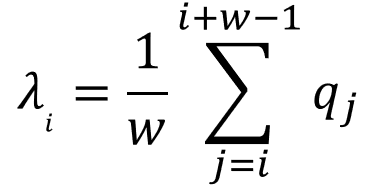The “linear charge density (LCD)” was defined as the sum of the charges over a given number (w, representing “window”) of consecutive amino acids in a single polypeptide chain, divided by w. For example, if the window width w = 20, then the linear charge density at index i (i is a positive integer) is the sum of the charges of the 20 amino acids from position i to position i+19 divided by 20. We arbitrarily chose w = 20. One of the justifications of choosing 20 was that the average alpha helix spanning through a membrane is roughly that long. The formula for the linear charge density at position i (λi) was given as the following:Where w is window width and qj is the average charge of the side chain of that residue at pH 7. We used −1 for aspartic acid and glutamic acid, and +1 for arginine and lysine, and +0.1 for histidine. Interestingly, the non-secreted proteins all had “peaks” over +2 (unit was e/20 aa, elementary charges per twenty amino acids) on more than one positions within its sequence, while almost all of the secreted proteins had no such peaks (and as specified in Discussions, these exceptions had their peaks mainly composed of lysines, not arginines). The LCD graphs were obtained using a script that we developed and published as a webserver.

#### Short Intro

Dr. Ahn's Microbial Engineering Lab